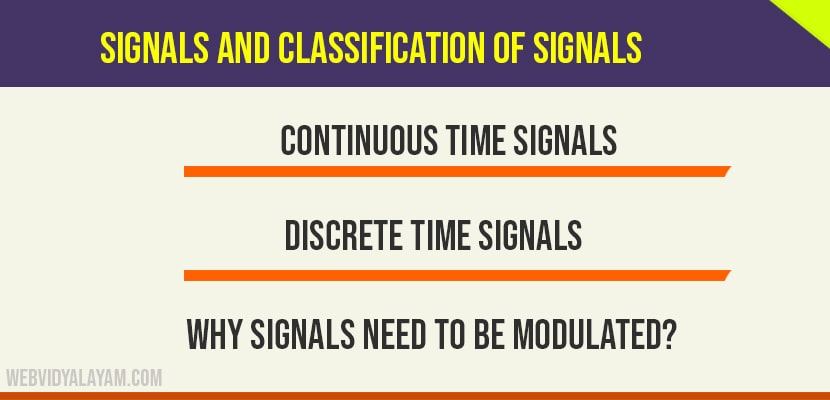# What are signals and classification of signals

## What are Signals

Anything that carries information is called signal. It may also be defined as any physical quantity that varies with time, space or any other independent variable. If signal depends only on one independent variable it is called one dimensional signal and if the signal depends on two independent variables then it is two dimensional signals. Signals may be represented in time or frequency domain.

## Classification of Signals

Signals can be classified in to two type and they are:

1. Continuous time signals
2. Discrete time signals

### Continuous Time Signals

Continuous time signals are defined for all instants of time. These are represented by x(t),t is an independent variable in time domain. Continuous time signals are represented by a function or graph.

### Discrete Time Signals

Discrete time signals(DTS) are defined only at discrete instants of time. These are represented by x(n).’n ’is an independent variable in time domain. There are four basic ways of representing DTS they are graphical, function, tabular and sequence.

### Attenuation

Attenuation is the loss in the strength of the signal or amplitude of the signals it travels through the channel. Noise, long distances causes the attenuation of the signal.

### Amplification

Amplification is the process of increasing the amplitude of the signal by the given factor.

### Bandwidth

Bandwidth is defined as the difference between upper and lower frequencies of the signal.

### Modulation

Modulation is the process of changing the characteristics of the carrier signal with respect to the original base band signal. There are different types of modulations Amplitude modulation, Frequency modulation and Phase modulation that we will discuss  them in detail further.

### Demodulation

Demodulation performs reversal operation of modulation. It retrieves the original message signal from the modulated signal using some demodulation schemes.

### Repeater

Repeater is used to strengthen the received  signal and retransmits it over long distances.

The terms transducer and receiver are explained in the previous topic.

## Why signals need to be modulated?

We know that baseband signals transmitted by the transmitter is received by the receiver. You might be having a question that when the baseband signals can be transmitted directly to the receiver then what is the need of modulation? Let us assume that if all the baseband signals are transmitted over a single transmitter then what happens is that all these signals are combined as they have the same frequency range. Due to this combining the information carried by those signals gets overlapped and sent to the receiver it is difficult to the receiver to separate these combined signals. Therefore each baseband signal has to be modulated for efficient transmission.

What is signal?

Anything that transmits information is called signal.

What are the 2 types of signals?

Continuous time and discrete time signals.

What is continuous time signal?

Continuous time signals are defined for all instants of time.

What is discrete time signals?

Discrete time signals(DTS) are defined only at discrete instants of time.